Disclaimer: This is an example of a student written essay.

Any scientific information contained within this essay should not be treated as fact, this content is to be used for educational purposes only and may contain factual inaccuracies or be out of date.

# Simultaneous Equilibria in the Benzoic Acid

 ✅ Paper Type: Free Essay ✅ Subject: Chemistry ✅ Wordcount: 2532 words ✅ Published: 1st Dec 2017

• Jekathjenani Ratnakumaran

Simultaneous Equilibria in the Benzoic Acid – Toulene -Water System

Introduction:

Benzoic acid is an aromatic carboxylic acid with molecular formula, C7H6O2. It appeared as colorless crystalline solid and has a fade, pleasant odor. Benzoic acid is easily available in nature and are used as a precursor to plasticizers, and sodium benzoate. Also, it inhibits the growth of microorganisms such as yeast, mold and bacteria. It also, used as an ointment to treat the fungal diseases such as ringworm and athlete’s foot. This benzoic acid can be prepared from the bromobenzene, benzyl alcohol and benzyl chloride.

If you need assistance with writing your essay, our professional essay writing service is here to help!

The purpose of this experiment is to determine the distribution coefficient (α) and dimerization constant of the benzoic acid in equilibrium of toluene and water. The distribution coefficient can be determined by using the distributive law which is given by the Nernst in 1891. This law states that distribution of the solute either solid or liquid between two non miscible solvents1. When the solute distributes in the two non miscible solvent at constant temperature, the solute remains in both solvent at the same molecular conditions and then, the concentration of solute in those two non miscible solvents will be equal to the distribution constant, K. In this experiment, the two non miscible solvents are water and toluene. There are three equilibrium states occur in the system used in this experiment such as distribution equilibrium, aqueous phase equilibrium and toluene phase equilibrium. These three equilibria contain benzoic acid (HBz), water (W) and Toluene (T). The distribution occurs as monomer in water and hence, there is monmer-dimer equilibrium occurs in the water and toluene phase2. From the distribution equilibrium, the distribution coefficient, α can be calculated by,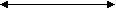HBz (w)HBz (T)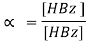From the aqueous equilibrium phase, the aqueous dissociation constant, Ka is calculated as follows,HBz (w)H+ + Bz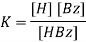From the toluene equilibrium phase, the aqueous dimerization constant, K2 is calculated as follows,2HBz (T)(HBz)2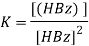The total concentration of benzoic acid in Toluene (CT) and total concentration of benzoic acid in water (Cw) can determine using the formula 1 and 3, they are as follows,

Cw= [HBz]w + [H+]

CT= [HBz]T + 2[(HBz)2]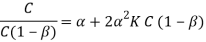By plotting the graph using above formula, the total concentrations present in both water and toluene can be determined.

Methods:

This experiment was conducted by following the procedure given in the laboratory manual by the department of chemistry, Trent University.

The structure of a dimer of benzoic acid is shown below,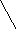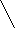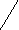O H- – – O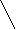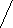C CO- – – H O

As benzoic acid has phenyl group and it is non polar but water is a polar solvent. Therefore, the carboxyl group in benzoic acid will be harder to form hydrogen bonding with water and decrease the interactions between the benzoic acid molecules. Hence it does not form a dimer with water. In the case of hexane, the hexane is non polar solvent and therefore, the benzoic acid forms dimer with hexane. In the case of methanol, as it has hydrogen bonding, it is considered as polar solvent. When it reacts with benzoic acid, it does not form a dimer of benzoic acid. Furthermore, in the case of propanone, benzoic acid does not form dimer because propanone is a polar solvent.

As the titrant solution is base solution, the indicator required to give end point is phenolphthalein. The indicator used in the titration is based on the pH values of the solutions used. In this case, as solution contains benzoic acid and its titrated against NaOH, therefore, the to bring the end point, the phenolphthalein which has pH approximately 9 is used. If the titrant is base solution, then the methyl orange, which has pH 3.5 is used to identify the end point.

Results:

Table 1 represents the volume of the benzoic acid, toluene and water added in the five solutions in different fractions of the volumes.

 Solutions Volume of benzoic acid in toluene Solution (ml) Volume of water added (ml) Volume of toluene required to bring total volume to 100 ml (ml) 1 50 50 0 2 40 50 10 3 25 50 25 4 15 50 35 5 5 50 45

Mass of the benzoic acid used = 5.000 g

Volume of the NaOH used= 10 ml

Calculated concentration of the NaOH = 0.018 M

Table 2 represents the titrated volume of the solutions against NaOH and HCl and calculated concentrations of benzoic acid in the Toluene and Water.

 Solution Number VNaOH (w) (ml) VNaOH(T) (mL) VHCl (T) (mL) CW x 10-3 (M) CT x 10-2 (M) β Cw (1-β) CT/Cw (1-β) 1 5.8 35.7 0.3 4.35 2.70 0.1141 0.00385 7.0130 2 5.4 28.9 0.4 4.05 2.20 0.1181 0.00357 6.1625 3 4.9 16.4 0.8 3.67 1.30 0.1236 0.00322 5.3450 4 3.0 10.7 1.6 2.25 0.92 0.1545 0.00190 4.8421 5 2.2 5.7 5.0 1.65 0.80 0.1773 0.00136 5.8823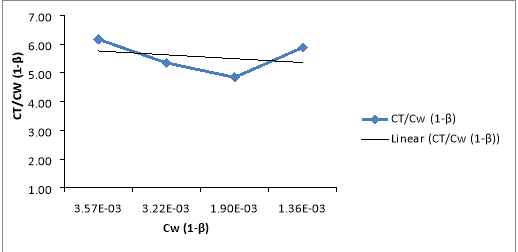Figure 1 represents the plot of Cw (1-β) versus CT/Cw (1-β).

Data Analysis & Calculations:

Volume of HBz in water, V1= 0.02 L

Volume of NaOH, V2= 0.0058 L

Concentration of NaOH, C2= 0.015 M

Concentration of HBz, Cw= (0.0058 x 0.015) / 0.02 M

= 4.35 x 10-3 M

Volume of HBz in water, V1= 0.02 L

Volume of NaOH + HCl, V2= 0.036 L

Concentration of NaOH, C2= 0.015 M

Concentration of HBz, CT= (0.036 x 0.015) / 0.02 M

= 2.70 x 10-2 M

By using the below formula, the value of β was calculated,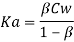The value of Ka for benzoic acid is 6.46 x 10-5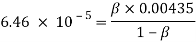0.00435 β2 + 6.46 x 10-5 β – 6.46 x 10-5

Using the quadratic formula, the value of β is,

β = 0.1141

Calculation of Cw (1-β) for solution 1,

Cw (1-β)= 0.00435 (1- 0.1141)

= 0.00385

Calculation of CT/Cw (1-β) for solution 1,

CT/Cw (1-β)= (0.0270) / (0.00385)

= 7.013

From the figure 1, the value of K2 and α are as follows,Y = -0.1344 x + 5.8939

α (intercept) = 0.1344

2K2= 5.8939

K2= (5.8939 ) / (0.03613)

= 163.13 M

Discussion:

The experiment was conducted with careful consideration to get the result as close as to the real value. However, due to the some experimental error involved in this experiment, the calculated values are not close to the literature value. The calculated K2 value is 163.13 L/mol and the alpha value is 0.1344. The literature values are 260 L/mol and 1.49 respectively. Figure 1 represents the plot of Cw (1-β) versus CT/Cw (1-β). Based on the graph shown on figure 1, the peak is falling and then raising. When the amount of benzoic acid decreases, the amount of concentration of toluene increases. On the contrary, the value of concentration of benzoic acid (HBz) in water is higher than the concentration of HBz in toluene. In comparison with the literature value, the calculated value has deviated from it. However, the calculated percentage error is 37 %. This is considered as large difference when compared to literature values. These differences between the calculated and literature value occurred due to the experimental error. Equipments used in this experiment were used by the previous batch. As the experiment was conducted using the pre used equipments, there might be an error in the contamination. The solution that has made in this experiment has to be mixed well. This will provide a more accurate result. Failure to do this step might have resulted in the failure of result. Also, the burette reading and end point notification are considered one of the errors which lead to inaccurate result. Due to these occurrences, the experiment does not turn successful and have heavily affected the final results. Moreover, the adjustment made in the water bath and less time of keeping the solution in the water bath also might be lead to the error in the final result. Because temperature is ne of the factor affects the dissociation of particles. Furthermore, due to the weather condition (winter), temperature and pressure changes and might not favored to conduct the experiment.

Conclusion:

In conclusion, the simultaneous equilibria of benzoic acid presence in the water and toluene solvent was studied by conducting this experiment. In order to get accuracy of result, the precision can be improved by more attention on the experimental procedure and handling the equipments. Also, accurate result can be made by observing the measurements such as notifying the burette reading and end point in a proper manner.

References

2. Ellison H.R. Simultaneous equilibria in the benzoic acid-benzene-water system, A distribution experiment; 48 (2): 124 (1971). DOI: 10.1021/ed048p124P.
3. Atkins and J. de Paula, Atkins’ Physical Chemistry, 8th ed., W. H.Freeman and Co., New York (2006).
4. Department of Chemistry, Laboratory Manual, Experiment G1- Heat Capacity Ratio for Gases, Trent University, Peterborough, Ontario(2014)

View all

## DMCA / Removal Request

If you are the original writer of this essay and no longer wish to have your work published on UKEssays.com then please:

Related Services

Related Lectures